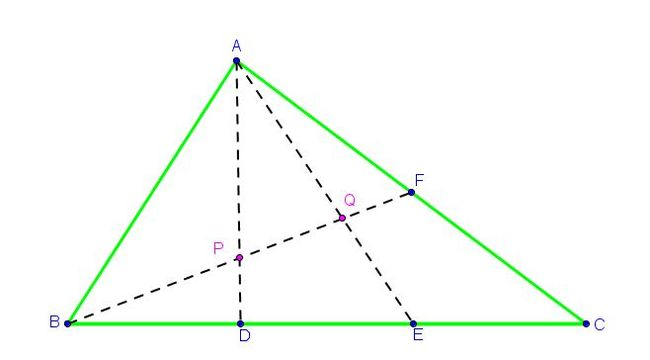# What name shall I give to this problem? - 2

Geometry Level 5In $\triangle ABC$, $D$ and $E$ are points on $BC$ such that $BD = DE = EC$. $F$ is a point on $AC$ such that $AF=FC$. If $AD$ and $AE$ intersect $BF$ respectively at $P$ and $Q$. Then, the ratio $\dfrac{BP}{PQ}$ can be expressed as $\dfrac{a}{b}$, where $a$ and $b$ are coprime integers. Find $10a+b$.

×